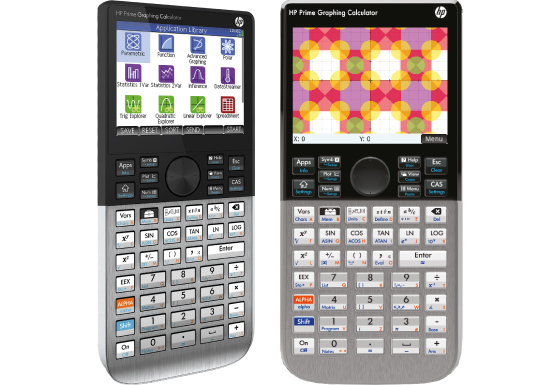## HP Prime G2

Graphing calculator HP Prime has a 3.5-inch full color touch screen, that delivers touch screen or keypad interaction. A range of advanced built-in and programmable functions, a rechargeable built-in long-life Li-Ion battery, easy way to switch between RPN, textbook and algebraic entry-system logic, an optional Wi-Fi adapter and more makes HP Prime the leading edge in graphing calculators.Specification

• Built-In Functions: 2300
• Entry System: Algebraic, RPN, textbook
• Total Memory Size: 32 MB, 256 MB Flash Memory
• Power Source: Rechargeable Li-Ion Battery
• Dimensions and Weight: 182 × 86 × 14 mm, 228 g

Functions

• Equation Editor
• Multi-Line Playback
• CAS Application (Computer Algebra System)
• EXAM Mode

Statistics

• 1-Variable/2-Variable Statistics
• Regression Calculations
• Scatter Plots and Histograms
• Inferential Statistics
• Probability Distribution

Graphing Functions

• Function Graphing
• Parametric Graphing
• Polar Graphing / Sequence Graphing
• Split Screen
• Function Table
• Slide Show

Mathematical Functions

• Logarithmic and Exponential Functions
• Trigonometric Functions
• Cyclometric and Hyperbolic Functions
• Factorial
• Random Numbers
• Fractions